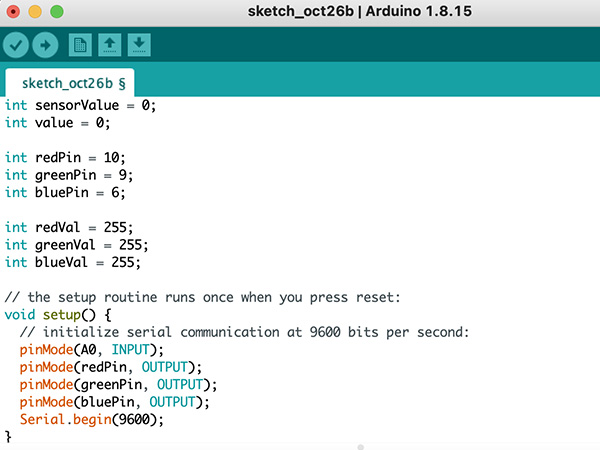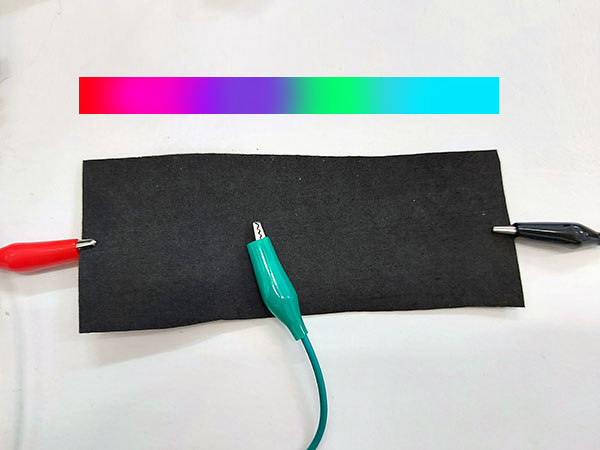# E-textiles

Engineers create a programmable fiber In a first, the digital fiber contains memory, temperature sensors, and a trained neural network program for inferring physical activity

New fibers can make breath-regulating garments “Robotic” textiles could help performers and athletes train their breathing, and potentially help patients recovering from postsurgery breathing changes.

### Analog Sensor

based on a project by Ana Correa Ana Correa Analog Tilt Sensor

### Circuit Prototype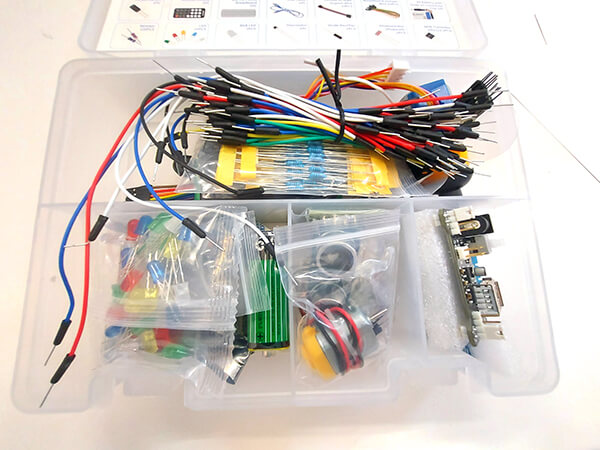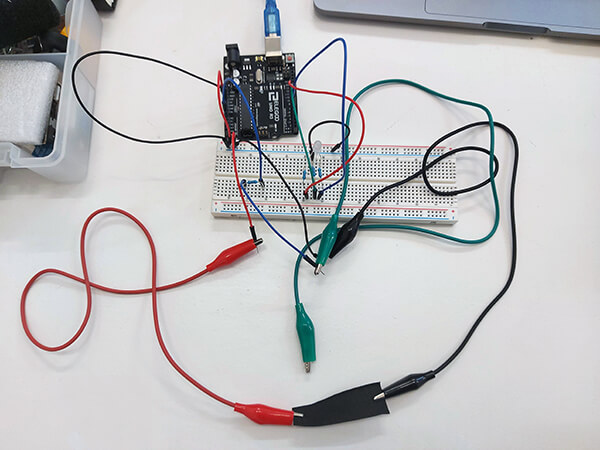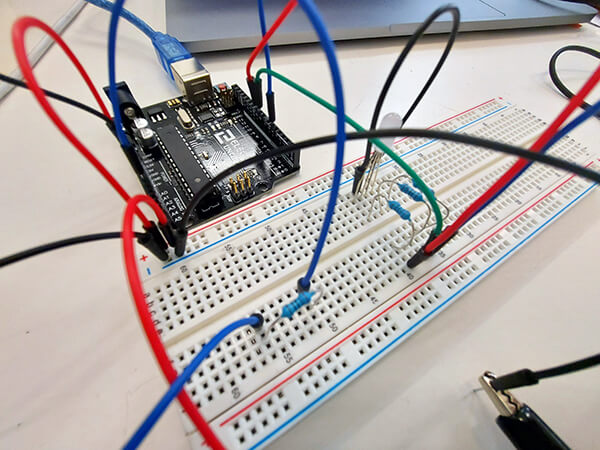### Code

`````` int sensorValue = 0;
int value = 0;

int redPin = 10;
int greenPin = 9;
int bluePin = 6;

int redVal = 255;
int greenVal = 255;
int blueVal = 255;

// the setup routine runs once when you press reset:
void setup() {
// initialize serial communication at 9600 bits per second:
pinMode(A0, INPUT);
pinMode(redPin, OUTPUT);
pinMode(greenPin, OUTPUT);
pinMode(bluePin, OUTPUT);
Serial.begin(9600);
}

// the loop routine runs over and over again forever:
void loop() {
// read the input on analog pin 0:
// print out the value you read:

sensorValue = constrain(sensorValue, 150, 400);

// Go from white to Cyan
if (sensorValue >= 0 && sensorValue <= 150);
{
int CyanColor = map (sensorValue, 0,150,0,255);
redVal = 255 - CyanColor;
greenVal = 255;
blueVal = 255;
// CyanColor rgb ( 0, 255, 255) 0 to 300
}

// Go from Cyan to Green
if(sensorValue > 150 && sensorValue <= 200){
int GreenColor = map(sensorValue, 200,300,0,255);
redVal = 0;
greenVal = 255;
blueVal = 255 - GreenColor;
// GreenColor  rgb(0,255,0)  300 -  450
}

// Go from Green to Yellow
if(sensorValue > 200 && sensorValue <= 250){
int YellowR = map(sensorValue, 300,400,0,255);
redVal = YellowR;
greenVal = 255;
blueVal = 0;
// yellow rgb(255,255,0) 450 to 600
}

// Go from Yellow to Magenta
if(sensorValue > 250 && sensorValue <= 300){
int MagentaR = map(sensorValue, 400,500,0,255);
redVal = 255;
greenVal = 255 - MagentaR;
blueVal = MagentaR;
// Magneta rgb(255,0,255) 600 to 750
}

// Go from Magenta to Red
if(sensorValue > 300 && sensorValue <= 350){
int RedR = map(sensorValue, 500,550,0,255);
redVal = 255;
greenVal = 0;
blueVal = 255 - RedR;
// Red rgb(255,0,0) 750 to 850
}

analogWrite(redPin, redVal);
analogWrite(greenPin, greenVal);
analogWrite(bluePin, blueVal);

Serial.print("sensorValue = ");Serial.print(sensorValue);Serial.print(" | ");
Serial.print("RedVal = ");Serial.print(redVal);Serial.print(" | ");
Serial.print("GreenVal = ");Serial.print(greenVal);Serial.print(" | ");
Serial.print("BlueVal = ");Serial.println(blueVal);

delay(10);        // delay in between reads for stability

}``````

### Input Values Range and Mapping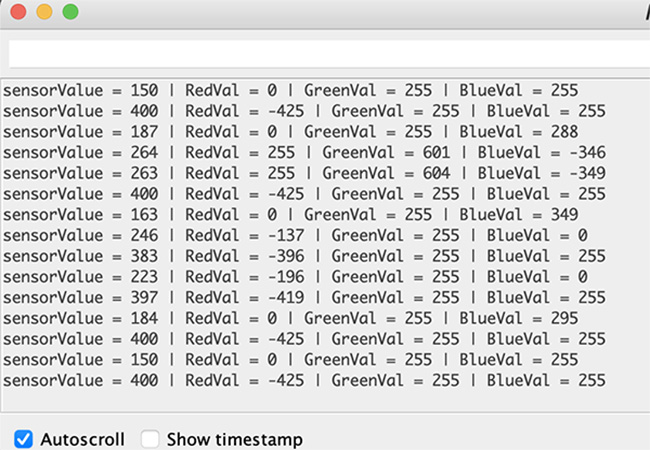The input data range was around 150-350. Therefore the output LED Diode light frequency was divided in 5 equal parts to map the color from red to cyan.